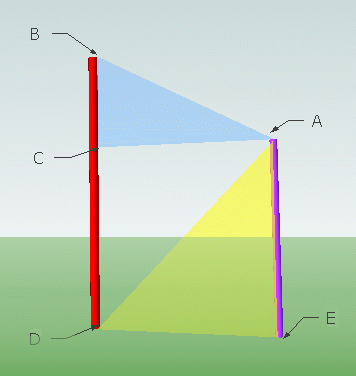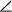SEARCH HOMEMath Central Quandaries & QueriesQuestion from joyce, a student: The angle of elevation of tower B from the top of tower A is 28 degrees and the angle of elevation of the top of tower A from the base is 46 degrees. Find the height of tower A if tower B is 120 m high?Hi Joyce.

I drew a diagram of the problem on the right.

We want AE.
We know DB = 120m,CBA = 28°,ADE = 46°.

First, note that AE = AB tan 46°. Also, AE = CD.

Now, note that BC = AC tan 28°. Also AC = AB.

Thus, DB = AB tan 46° + AB tan 28° = 120m.

Solve for AB. Use this to solve for AE in our earlier equation.

Hope this helps,
Stephen La Rocque.Math Central is supported by the University of Regina and The Pacific Institute for the Mathematical Sciences.